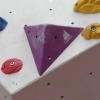Search by Topic

Resources tagged with Vector algebra similar to Complex Rotations:

Filter by: Content type:
Age range:
Challenge level:

There are 7 results

Broad Topics > Vectors and Matrices > Vector algebraThree by One

Age 16 to 18 Challenge Level:

There are many different methods to solve this geometrical problem - how many can you find?Tetra Perp

Age 16 to 18 Challenge Level:

Show that the edges AD and BC of a tetrahedron ABCD are mutually perpendicular when: AB²+CD² = AC²+BD².Quaternions and Rotations

Age 16 to 18 Challenge Level:

Find out how the quaternion function G(v) = qvq^-1 gives a simple algebraic method for working with rotations in 3-space.Quaternions and Reflections

Age 16 to 18 Challenge Level:

See how 4 dimensional quaternions involve vectors in 3-space and how the quaternion function F(v) = nvn gives a simple algebraic method of working with reflections in planes in 3-space.Age 16 to 18 Challenge Level:

A quadrilateral changes shape with the edge lengths constant. Show the scalar product of the diagonals is constant. If the diagonals are perpendicular in one position are they always perpendicular?